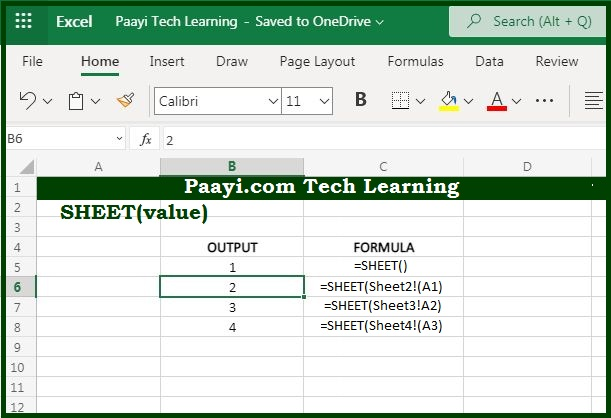# Learn How to Use Microsoft Excel SHEET Function

Written by | 0 Comments | 736 Views

In this article, you will learn how to use the Microsoft Excel SHEET function and its prime function in Microsoft Excel. You will also get to know the Microsoft Excel SHEET function return value and syntax with the help of some examples.

Microsoft Excel SHEET Function

The main use of the Microsoft Excel SHEET Function is to get the index number of the sheet. So, you can able to return the index number of excel in Microsoft Excel. The SHEET function will report the sheet number for a cell reference, named range or Sheets table.

Return Value of SHEET Function

The return value will be the index number of the given sheet.

Syntax of SHEET Function

=SHEET([value] )

Where the arguments:

value: It is the value to check (optional).

## How to Use Microsoft Excel SHEET Function?You can use the SHEET function to get the index number for a given sheet. The index number represents the numeric sequence of sheets in the workbook, it starts with 1 on the left and ends with N on the left. Where N is the total number of sheets in the workbook. The SHEET function includes hidden sheets in the numbering sequence.

It should be noted that:

The SHEET function includes hidden sheets in the numbering sequence.

In case the value argument is not present, SHEET will return the index of the sheet it's entered on.

Usually, the value is provided as a cell reference, but you can also supply a named range or the name of an Excel Table

The SHEET function reports the index of a sheet, the SHEETS function reports the number of sheets in a reference.